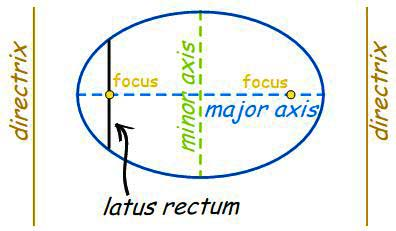## Conic Sections

Definition:
The curves obtained by intersection of a plane and a double cone in different orientation are called conic section.### Definitions of various important terms:

1. Focus: The fixed point is called the focus of the conic-section.2. Directrix: The fixed straight line is called the directrix of the conic section.
3. Eccentricity: The constant ratio is called the eccentricity of the conic section and is denoted by e.4. Axis: The straight line passing through the focus and perpendicular to the directrix is called the axis of the conic section. A conic is always symmetric about its axis.
5. Vertex: The points of intersection of the conic section and the axis are called vertices of conic section.
6. Centre: The point which bisects every chord of the conic passing through it, is called the centre of conic.
7. Latus-rectum: The latus-rectum of a conic is the chord passing through the focus and perpendicular to the axis.8. Double ordinate: The double ordinate of a conic is a chord perpendicular to the axis.
9. Focal chord: A chord passing through the focus of the conic is called a focal chord.
10. Focal distance: The distance of any point on the conic from the focus is called the focal distance of the point.

### General equation of a conic section when its focus, directrix and eccentricity are given:

Let S(α, β) be the focus, Ax + By + C = 0 be the directrix and e be the eccentricity of a conic.Let P(h, k) be any point on the conic. Let PM be the perpendicular from P, on the directrix. Then by definition,
SP = ePM ⇒ SP2 = e2PM2### Recognisation of conics

The equation of conics is represented by the general equation of second degree ax2 + 2hxy + by2 + 2gx + 2fy + c = 0 ……(i)
and discriminant of above equation is represented by ∆, where ∆ = abc + 2fgh – af2 – bg2 – ch2
Case I: When ∆ = 0.
In this case equation (i) represents the degenerate conic whose nature is given in the following table.

 S. No. Condition Nature of conic 1. ∆ = 0 and ab – h2 = 0 A pair of coincident straight lines 2. ∆ = 0 and ab – h2 < 0 A pair of intersecting straight lines 3. ∆ = 0 and ab – h2 > 0 A point

Case II: When ∆ ≠ 0.
In this case equation (i) represents the non-degenerate conic whose nature is given in the following table.

 S. No. Condition Nature of conic 1. ∆ ≠ 0, h = 0, a = b, e = 0 A circle 2. ∆ ≠ 0, ab – h2 = 0, e = 1 A parabola 3. ∆ ≠ 0, ab – h2 > 0, e < 0 An ellipse 4. ∆ ≠ 0, ab – h2 < 0, e > 0 A hyperbola 5. ∆ ≠ 0, ab – h2 < 0, a + b = 0, e = √2 A rectangular hyperbola